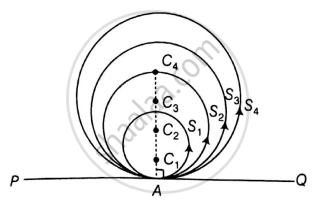# . If a number of circles touch a given line segment PQ at a point A, then their centres lie on the perpendicular bisector of PQ. - Mathematics

MCQ
True or False

If a number of circles touch a given line segment PQ at a point A, then their centres lie on the perpendicular bisector of PQ.

• True

• False

#### Solution

This statement is False.

Explanation:Let the S1, S2, S3, …., Sn be n circles with centers C1, C2, C3, …, Cn respectively.

And The PQ is a common tangent to all the circles at point A which is common to all circles.

As we know,

Tangent at any point on the circle is perpendicular to the radius through point of contact

We have,

C1A ⏊ PQ

C2A ⏊ PQ

C3A ⏊ PQ

CnA ⏊ PQ

So, C1, C2, C3 … Cn all lie on the perpendicular line to PQ but not on perpendicular bisector as

PA may or may not be equal to AQ.

Concept: Concept of Circle - Centre, Radius, Diameter, Arc, Sector, Chord, Segment, Semicircle, Circumference, Interior and Exterior, Concentric Circles
Is there an error in this question or solution?

#### APPEARS IN

NCERT Mathematics Exemplar Class 10
Chapter 9 Circles
Exercise 9.2 | Q 8 | Page 106
Share